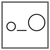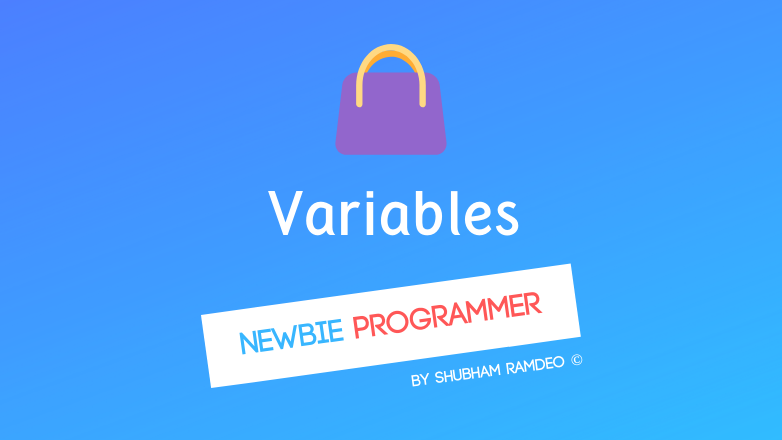Fuddlepixel coz tech is fun();

# Variables a handbag to store data!

Lets discuss the concept of storing data into memory bags called variables. What are variables and how to use them and add comments in you codes in C ?

By Shubham Ramdeo
06 July 2015 · 7 mins read
# `c`  `newbie`Lets put some numbers into the bags of memory called Variables !

Welcome to the Newbie Programmer series. The last post was interesting as we have started the real coding. But this one is even more. In this part, we learn about variables. What is a variable and What is their use. If you are new to this series, please go here and read all the earlier parts so that you can easily understand what is going on.

So Variables. Women can understand this more easily than men. The variable is a kind of handbag. That’s what I learned, It’s a handbag that stores certain things. We give names to these handbags and call them, in mathematics, a variable.

Also, women use different kinds of handbags for storing different kinds of things. Like one for clothes, one for their jewelry.

Let us Consider these following points for a woman.

• She has a bag.
• She call her bag “clothBag”
• At present, the bag is containing a Tunic.

Now based on the above 4 points, Can I write something like this

``````Bag clothBag;
clothBag = Tunic;
``````
• 1st line means, there is a bag called “clothBag” which is used for storing clothes.
• 2nd line means that the “clothBag” bag is currently containing a Tunic.

And hence we have made the above 3 points in a coding style.

That’s it. Variables ends here. There is no more, nothing to learn about them. Variable is a bag, it has its type, means what type of things it can store, and has certain thing presently stored in it.

### But what is the use of variables ?

Well, what is the use of a handbag ? we store things in it for portability and can even add or remove the things inside the bag. similarly with variables, the word itself means “changeable”.

Now let’s do a little maths and let the girls keep their handbags. Here begin the real variable use for us.

In maths, we have a number system called Integer. Integer means a complete negative or positive Number or zero. Don’t think hard. -1, -3, 5, 7, 0, 10, 22, 45 etc.. all these simple numbers from 0 to infinity in both positive and negative sides is an integer. So -1532 and 75729 whatever is an integer but 11/4, 1/2, pi, 5/4, -22/5, root 2.. such numbers are fractions and not complete so these are not integers.

Now I wanna make a variable whose name is x, it stores only integers, and presently, x is 4. So I would say :

``````int x;
x = 4;
``````

Let’s do one more,

``````int y;
y = 3;
``````

And what would be this one ?

``````int z;
z = x + y;
``````

z is a variable that can contain integers inside it. At present, z = x + y which means 3 + 4 as written above so now, z = 7.

### Assignment vs Equality

Now I am gonna show you a (mind-blowing) thing that every non-programmer mathematician asks me !

What is a = a + 1 ?

This thing : a = a + 1 is absolutely Incorrect in maths !

But in programming, it’s no problem.

Like this:

``````int a;
a = 3;
a = a + 1;
``````

Now can you say what is ‘a’ ? In programming, the ‘ = ‘ sign not simply means that right and left side should be equal, It means that “Now the left side contains the value of Right.”

So we know that a is an integer. ‘a’ value is 3. Then in last line, a = a + 1, apply Now the left side contains the value of Right. So a contains the value of a + 1, and we know a is 3 so again a contains the value of 3 + 1, Or finally, a is 4.

Hence this piece,

``````int a;
a = 3;
a = a + 1;
``````

brings final value of a is 4.

Q. Can you predict the value of z in this following case ?

``````int x;
int y;
int z;

x = 4;
y = x + 2;
z = y + x;
z = z + 2;
z = ???
``````

Ans: z = 12

You can do simple reverse engineering, go upward in the above code.

``````z = z + 2
z = [y + x] + 2
z = [(x + 2) + x ] + 2

And we have written above x = 4 so substitute,

z = [(4 + 2) + 4] + 2
z = [6 + 4] + 2
z = 10 + 2
z = 12
``````

That’s it.

## Using Variables in C

For now, we will consider only integers. So in C programming language, to create a integer variable, simply use this command:

``````int <variable name>;
``````

like :

``````int marks;
``````

to provide any value to that variable

``````<variable name> = <value>;
``````

like :

``````marks = 90;
``````

or you can do it all together :

``````int marks = 90;
``````

Never forget to put (;) after every instruction. These are like full stops for coding.

### Printing Numbers

We have learned you to use printf command from the previous post. Here We would extend its use.

``````printf(" whatever to be shown on screen ", list of variables );
``````

And to show integer we will put, %d just like we put /n for new line. We will discuss this stuff in detail later.

So now check out the following program:

``````#include <stdio.h>
main()
{
int a = 10;
int b = 2;
int c = a + b;
printf("Sum of a and b is %d", c);
}
``````

OUTPUT :

``````Sum of a and b is 12
``````

If you change the values of a and b, sum will also change. And that is why we use variables so that We just change the values inside variables and all logic remains the same.

### More maths ?

Learn to use these operations in C for maths.

• a + b to add a and b
• a – b to subtract a and b
• a * b to multiply a and b
• a / b to divide a by b

We can also put comments inside the code. Every thing written between /* and / will be skipped by the C language. So in any piece of code we have ever written, put any song lyrics, abusive words whatever you want like this / I love programming */ will create no change.

Also you can use // to make the complete line a comment. The whole sentence after // will be skipped. So we can do // I love programming but then cannot write any further code in the same line.

### Let’s make a little calculator :

``````#include <stdio.h>
main()
{
int a = 1; // give your value to a
int b = 1; // give your value to b but not 0
/* Division by zero is not possible.
Program will not work if b = 0 !!! */

int sum = a + b;
int sub = a - b;
int multy = a * b;
int div = a / b;
printf("Sum is %d \n", sum);
printf("Subtraction is %d \n", sub);
printf("Multiplication is %d \n", multy);
printf("Division is %d \n", div);
}
``````

Change the values of a and b based on your calculation and then run the program. But never put b = 0 as division by zero is not possible and the program will not run. Please read this previous post (click here) to learn how to run a program.

That’s enough for today. We have learned one of the most important concepts in programming specially how a = a + 1 ! In the next post, we will do some awesome applications just like the calculator we have made above. So please stay connected.

##### Update me weekly ✉

Subscribe below and we’ll send you a weekly email summary of all new Code tutorials. Never miss out on learning about the next big thing.

#### Shubham RamdeoShubham Ramdeo is the founder of Fuddlepixel. He is a geek, a writer, involved with many technical and humanity projects.

Meet me!

#### Recent#### Related

`c`

`newbie`

Top